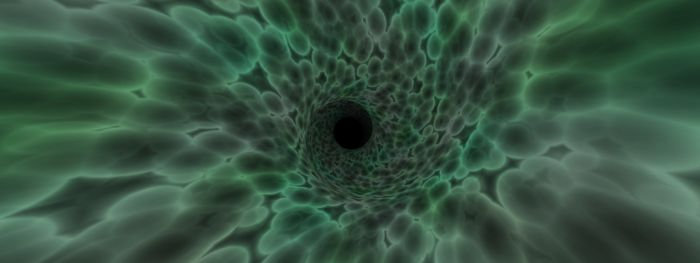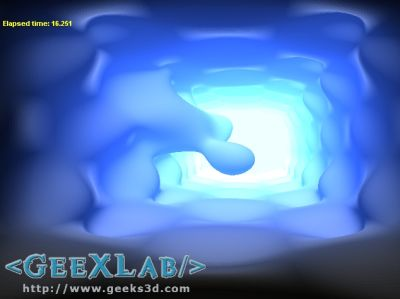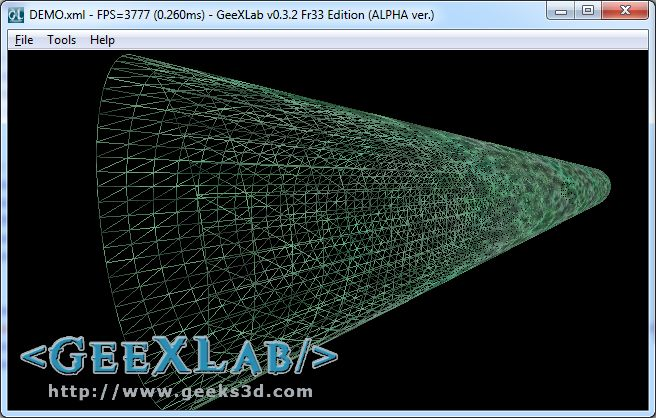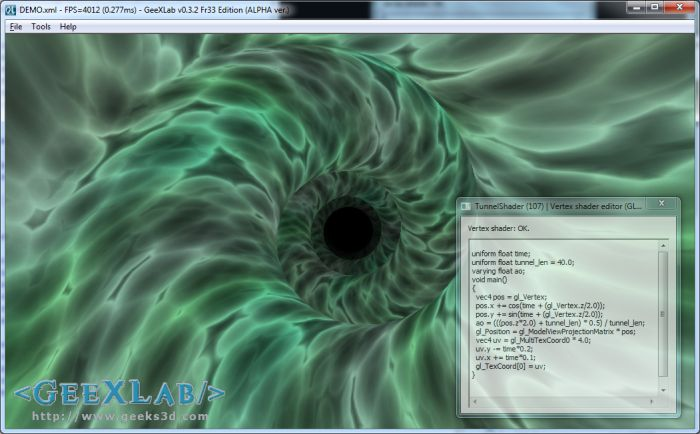# How to Make a Simple Tunnel EffectTunnel is a cool effect in graphics programming. You can do a tunnel effect using a real geometry (a mesh) or using procedural / ray tracing techniques like in this intro coded by XT95^FRequency (you can see this pixel shader based tunnel in action in ShaderToyMark):Metatunnel effect by XT95^FRequency

Today, I’m going to show you a simple mesh-based tunnel effect. The GeeXlab demo is based on this WebGL article.

 The GeeXLab demo is available in the code sample pack in the GLSL_Tunnel/ folder: [download#40#image] This demo requires GeeXLab 0.2.10 or better GeeXLab 0.3.0+* in order to play with GLSL live coding. *: Both GeeXLab 0.3.0 and 0.3.1 have a minor bug: the max width and height are clamped to 1024 pixels. It’s already fixed in the next v0.3.2…

The principle of this tunnel effect is quite simple: create a mesh cylinder and use a vertex shader to deform and animate the mesh.

1/ Create a mesh cylinder like this one:2/ Use the following GLSL program to deform and animate the cylinder:

```[Vertex_Shader]
uniform float time;
uniform float tunnel_len; // 80.0
uniform vec2 z_divisor; // 10.0, 10.0
varying float ao;
void main()
{
vec4 pos = gl_Vertex;
pos.x += cos(time + (gl_Vertex.z/z_divisor.x));
pos.y += sin(time + (gl_Vertex.z/z_divisor.y));
ao = (((pos.z*2.0) + tunnel_len) * 0.5) / tunnel_len;
gl_Position = gl_ModelViewProjectionMatrix * pos;
vec4 uv = gl_MultiTexCoord0 * 4.0;
uv.y -= time*0.2;
uv.x += time*0.1;
gl_TexCoord = uv;
}

uniform sampler2D tex0;
varying float ao;
void main (void)
{
vec2 uv = gl_TexCoord.xy;
vec3 t = texture2D(tex0,uv).rgb * ao;
gl_FragColor = vec4(t, 1.0);
}
```

The z_divisor uniform variable allows to get some cool deformations like this one:## 4 thoughts on “How to Make a Simple Tunnel Effect”

1.Mulata

YEEAH! Remember the days, when they used move-lists/tables?
Coming up next: roto-zoom and raster-bars.

2.xoofx

It is XT95 from FRequency not TX95 😉

3.JeGX Post Author

@xoofx: what a shame! Fixed now 😉 Ya que moi pour faire une erreur sur un nick aussi simple!

4.LLB

I think the oldschool way to do this effect is to do it in 2D, like in the shadertoy “Tunnel” example.